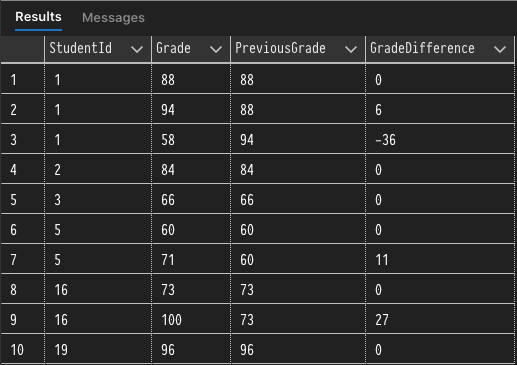# Learning T-SQL Window Functions

## Being able to query and manipulate data effectively is a valuable skill for any software engineer to have in their toolbelt. In this post, we're going to take a look at Window Functions and the functionality they provide. Although this post is going to focus on using window functions with T-SQL, it's important to note that window functions are part of the ANSI SQL standard and are available in other SQL database implementations.

Aaron Bos | Wednesday, January 19, 2022

## What Are Window Functions?

At a high level window functions allow us to reason about and perform calculations on small sets of data within a larger query. They are useful for doing statistical calculations like running totals and moving averages. Initially, window functions might seem very similar to aggregate functions, but they are quite different. Window functions use values from one or many rows to return a value for each row, whereas aggregate functions return a single value for one or many rows.

Here is a quick example demonstrating the difference between window functions and aggregate functions. While the syntax of the window function may not be familiar yet, I think the explanation and query result will be helpful in understanding key differences.

This first query is a straightforward aggregate query that groups on the CustomerID column and provides an aggregate sum of TotalDue for each group of CustomerIDs.

``````-- Aggregate SUM() Function
SELECT SUM(TotalDue), CustomerID
GROUP BY CustomerID
ORDER BY CustomerID;
``````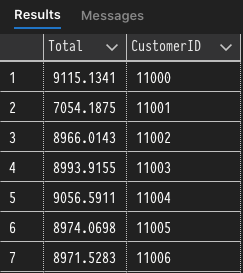The second query is very similar to the previous, but it includes the `ROW_NUMBER()` function that effectively numbers each row in the "window" defined by the `OVER` clause. It's key to note here that `ROW_NUMBER` is not constrained by the GROUP BY aggregation because it is evaluated afterward, so each row in the entire result set is numbered individually.

``````-- Window Function using ROW_NUMBER() and OVER
SELECT SUM(TotalDue), CustomerID,
ROW_NUMBER() OVER(ORDER BY CustomerID) as RowNumber
GROUP BY CustomerID;
``````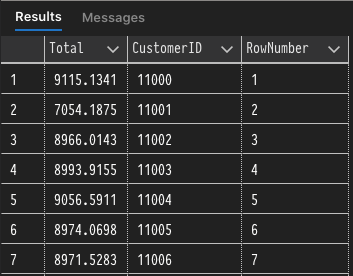Now that we've seen an example of a window function let's jump into the syntax used to define them in queries.

## Window Function Syntax

If you look at the documentation page for clauses involved in window functions it may seem a little overwhelming with all of the different options available. Personally, I think that the majority of uses cases for window functions can be broken down into four parts.

1. Function: performs calculations on the specified "window" of data
2. `OVER`: used to define the "window"
3. `PARTITION BY`: part of the `OVER` clause used to divide the result set into partitions
4. `ORDER BY`: an optional piece of the `OVER` clause which logically orders results in each "window"

We will look at the different kinds of window functions in the next section. First, we'll be placing our focus on the syntax and purpose of the `OVER` clause when used with `PARTITION BY` and `ORDER BY`.

The general syntax when using the `OVER` clause is relatively simple. We use `OVER` in conjunction with a function like `ROW_NUMBER()` to partition, order, and perform calculations against windows of data.

``````ROW_NUMBER() OVER([PARTITION BY expression] [ORDER BY expression])
``````

In the example below we can use the ROW_NUMBER function to apply a number to each row in the partitions defined by `PARTITION BY CustomerId` and ordered with `ORDER BY OrderDate`. As we can see from the result set below the query, the ROW_NUMBER function is applied to each partition of CustomerIDs and it starts over with each new partition (CustomerId). The rows within each partition are ordered by the OrderDate, which is defined within the `OVER` clause and not the overall query.

``````SELECT SalesOrderID, OrderDate, CustomerID,
ROW_NUMBER() OVER(PARTITION BY CustomerId ORDER BY OrderDate) AS RowNum
ORDER BY CustomerID;
``````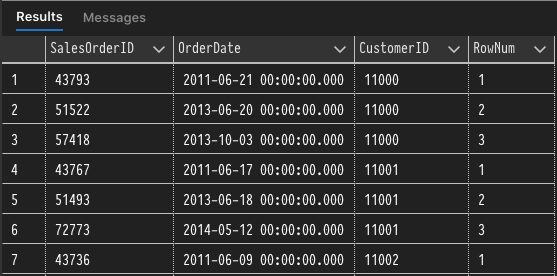Now that we've gone over the common syntax for window functions covered let's take a look at the different types of Window Functions and what they can be used for.

## Types of Window Functions

So far we've looked at the purpose and general syntax used with window functions, but we haven't talked specifically about the different kinds of window functions and what they can be used for. In the next three sections, we'll be looking at Ranking, Aggregate, and Analytic functions which can all be applied as window functions. First up is ranking functions.

### Ranking Functions

At a high level, the ranking functions return a ranking value for each row in a result set partition. We've already seen a couple of examples of a ranking function with `ROW_NUMBER`, which returns the sequential number of a row in a partition. The other ranking functions available to use are `RANK`, `DENSE_RANK`, and `NTILE`.

The `RANK` function will rank each row in a result set partition with a numeric value. `RANK` is very similar to `ROW_NUMBER` except that `RANK` will return the same number for ties. When ranking the `RANK` function will take into account both the `PARTITION BY` and `ORDER BY` column values. If we want to rank the entire result set instead of individual partitions, we can exclude the `PARTITION BY` clause.

Run the following code to test out the `RANK` function. As you'll see from the result set rows with Id 2 and 3 have the same quantity, which leads to an equal rank. An important thing to note is that gap is left in the rankings where 3 should be leaving the ordering to be `1,2,2,4,...`.

``````CREATE TABLE #RankTest (Id INT PRIMARY KEY NOT NULL, Quantity INT NOT NULL);
GO
INSERT INTO #RankTest (Id, Quantity)
VALUES(1, 0),(2,1),(3,1),(4,2),(5,3),(6,5);

SELECT Id, Quantity,
RANK() OVER(ORDER BY Quantity) AS QuantityRank
FROM #RankTest;

DROP TABLE #RankTest;
``````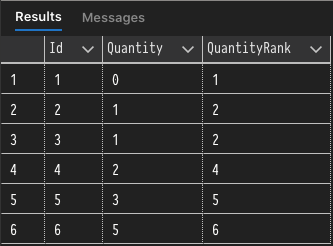`DENSE_RANK` is very similar to `RANK`, except that it does not leave a gap in the rankings. So if we use our previous example code, but call `DENSE_RANK`instead we'll notice that the resulting `QuantityRank` is `1,2,2,3,4...`.

``````CREATE TABLE #RankTest (Id INT PRIMARY KEY NOT NULL, Quantity INT NOT NULL);
GO
INSERT INTO #RankTest (Id, Quantity)
VALUES(1, 0),(2,1),(3,1),(4,2),(5,3),(6,5);

SELECT Id, Quantity,
DENSE_RANK() OVER(ORDER BY Quantity) AS QuantityRank
FROM #RankTest;

DROP TABLE #RankTest;
``````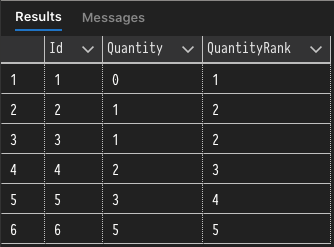`NTILE`, the last of the ranking functions to cover, is a little different in comparison to other ranking functions in that it is used to distribute rows in an ordered partition to different buckets. In the previous examples, the `ORDER BY` clause was optional, but with `NTILE` it is required in order to properly distribute rows within a partition. The best example I can think of for this function is a grading system that split results into quartiles (4 buckets). In the example below the grades will be distributed into 4 buckets (quartiles) based on the descending order of student grades.

``````CREATE TABLE #StudentGrades(Id int primary key not null, Grade int not null);
GO

VALUES(1,88),(2,66),(3,94),(4,60),(5,100),(6,84),(7,71),(8,96),(9,73),(10,58);

NTILE(4) OVER(ORDER BY Grade DESC) AS Quartile

``````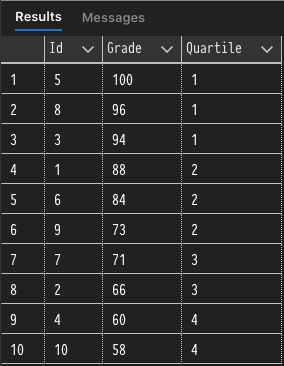### Aggregate Functions

The aggregate functions used with the `OVER` clause are the same aggregate functions that are commonly used when grouping data with `GROUP BY`. This includes functions like `MIN`, `MAX`, `AVG` and the rest. The only aggregate functions that can't be used as window functions are `STRING_AGG`, `GROUPING`, and `GROUPING_ID`.

Let's continue with the student grading example by introducing a `StudentId` column and multiple test scores for each student. We will then use the aggregate functions with `OVER` to return the `MIN`, `MAX`, `AVG`, and `COUNT` for each student.

``````CREATE TABLE #StudentGrades(Id int primary key not null, StudentId int not null, Grade int not null);
GO

VALUES(1,1,88),(2,3,66),(3,1,94),(4,5,60),(5,16,100),(6,2,84),(7,5,71),(8,19,96),(9,16,73),(10,1,58);

SELECT DISTINCT StudentId,
COUNT(Grade) OVER(PARTITION BY StudentId ORDER BY StudentId) AS TestsTaken

``````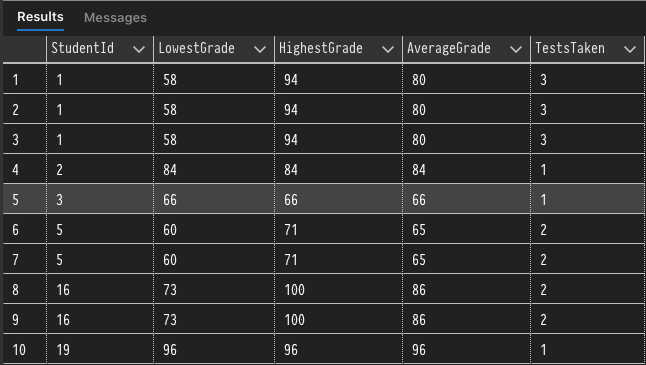While this could have also been accomplished by grouping, it's good to always have other options when grouping may not be the best option for the task at hand.

### Analytic Functions

Analytic functions are the final type of window functions that we'll be covering. They are used to calculate an aggregate value based on a group of rows. The main difference between aggregate and analytic functions is that analytic functions can return multiple rows for each group whereas aggregate functions will return a single row.

I don't plan on going through all of the analytic functions because they are relatively similar in functionality and self-explanatory, but I do think it would be worth showing an example for the `LAG` function. For the full list of analytic functions, check out the documentation here.

I find `LAG` to be an interesting function (`LEAD` is a very similar) because it actually looks at the previous row(s) to calculate a value. When using `LAG` we must use `ORDER BY` in our `OVER` clause and it has the following parameters.

1. `scaler_expression`: value to be returned based on specified offset.

2. `offset`: the number of rows back from the current row to obtain a value.

3. `default`: value returned when the offset is beyond scope of the partition.

When using the `LAG` function the first row of a partition will use the default value because there are no previous rows. For example, we can look at multiple grades for a student and see trends in how grades change over time. You'll notice that we use the `Grade` as the default value to avoid skewing the difference column when a partition begins.

``````CREATE TABLE #StudentGrades(Id int primary key not null, StudentId int not null, Grade int not null);
GO

VALUES(1,1,88),(2,3,66),(3,1,94),(4,5,60),(5,16,100),(6,2,84),(7,5,71),(8,19,96),(9,16,73),(10,1,58);